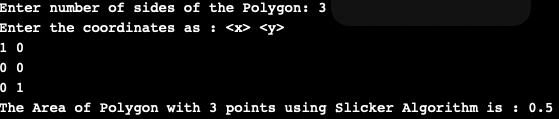# Slicker Algorithm to Find the Area of a Polygon in Java

• Difficulty Level : Basic
• Last Updated : 05 Nov, 2020

Slicker Algorithm is a way to determine the area of the n-sided polygon. This algorithm takes the y-direction pointing upwards as positive according to the mathematical conventions but according to computer systems where positive y-direction is downwards, the most efficient way is to list the vertices counter-clockwise using the positive y down coordinates which cancels out the two effects and in turn returns the positive area.

Example

```Input:
Enter number of sides of the Polygon: 4
Enter the coordinates as : <x> <y>
0 0
1 0
1 1
0 1
Output:
The Area of Polygon with 4 points using Slicker Algorithm is: 1      ```

Approach

```Take no of sides and coordinates of n-sided polygon as an input from the user

Define function Area() which calculates the area with p as an argument as follows:
for i = 0 to (p.n-1)
j = (i + 1) % p.n;
calculate area += (p.p[i].x * p.p[j].y) - (p.p[j].x * p.p[i].y);
print total area as area/2
```

Note: Input points must take in order, Program will not work properly with points taken in random order.

Below is the implementation of the above program

## Java

 `// Implement Slicker Algorithm that avoids``// Triangulation to Find Area of a Polygon``import` `java.util.*;``class` `Main {``    ``// defining the maximum no of sides for the Polygon``    ``static` `final` `int` `MAXSIDES = ``200``;`` ` `    ``static` `class` `Corner {``        ``double` `x, y;``    ``}`` ` `    ``static` `class` `Polygon {``        ``Corner p[] = ``new` `Corner[MAXSIDES];``        ``int` `n;`` ` `        ``Polygon()``        ``{``            ``for` `(``int` `i = ``0``; i < MAXSIDES; i++)``                ``p[i] = ``new` `Corner();``        ``}``    ``}`` ` `    ``// calculating area with Slicker Algorithm``    ``static` `double` `area(Polygon p)``    ``{``        ``double` `total = ``0``;``        ``for` `(``int` `i = ``0``; i < p.n; i++) {``            ``int` `j = (i + ``1``) % p.n;``            ``total += (p.p[i].x * p.p[j].y)``                     ``- (p.p[j].x * p.p[i].y);``        ``}``        ``return` `total / ``2``;``    ``}`` ` `    ``static` `public` `void` `main(String[] args)``    ``{``        ``Polygon p = ``new` `Polygon();`` ` `        ``Scanner sc = ``new` `Scanner(System.in);`` ` `        ``// Taking inputs from the user`` ` `        ``System.out.print(``            ``"Enter number of sides of the Polygon: "``);`` ` `        ``p.n = sc.nextInt();`` ` `        ``System.out.println(``            ``"Enter the coordinates as : "``);`` ` `        ``// Taking the coordinates of each Corner``        ``for` `(``int` `i = ``0``; i < p.n; i++) {``            ``p.p[i].x = sc.nextDouble();``            ``p.p[i].y = sc.nextDouble();``        ``}``        ``double` `area = area(p);``        ``if` `(area > ``0``)``            ``System.out.print(``                ``"The Area of Polygon with "` `+ p.n``                ``+ ``" points using Slicker Algorithm is : "``                ``+ area);``        ``else``            ``System.out.print(``                ``"The Area of Polygon with "` `+ p.n``                ``+ ``" points using Slicker Algorithm is : "``                ``+ (area * -``1``));``        ``sc.close();``    ``}``}`

Output:Time Complexity: O(N), Where N is a number of sides of the polygon.

My Personal Notes arrow_drop_up